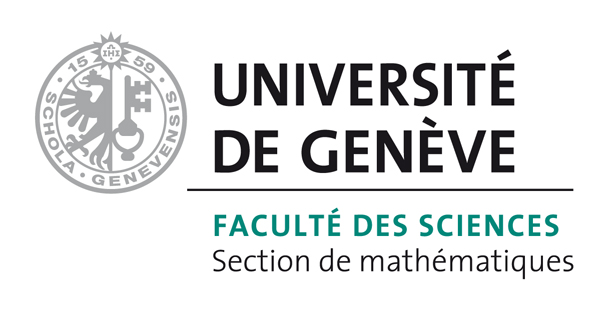# Bart Vandereycken## Low-rank matrix completion by Riemannian optimization

by

#### Abstract:

The matrix completion problem consists of finding or approximating a low-rank matrix based on a few samples of this matrix. We propose a new algorithm for matrix completion that minimizes the least-square distance on the sampling set over the Riemannian manifold of fixed-rank matrices. The algorithm is an adaptation of classical nonlinear conjugate gradients, developed within the framework of retraction-based optimization on manifolds. We describe all the necessary objects from differential geometry necessary to perform optimization over this low-rank matrix manifold, seen as a submanifold embedded in the space of matrices. In particular, we describe how metric projection can be used as retraction and how vector transport lets us obtain the conjugate search directions. Finally, we prove convergence of a regularized version of our algorithm under the assumption that the restricted isometry property holds for incoherent matrices throughout the iterations. The numerical experiments indicate that our approach scales very well for large-scale problems and compares favorably with the state-of-the-art, while outperforming most existing solvers.

#### Reference:

B. Vandereycken, "Low-rank matrix completion by Riemannian optimization", In SIAM J. Optim., vol. 23, no. 2, pp. 1214-1236, 2013.

#### Bibtex Entry:

@article{Vandereycken_2013,
Abstract = {The matrix completion problem consists of finding or approximating a low-rank matrix based on a few samples of this matrix. We propose a new algorithm for matrix completion that minimizes the least-square distance on the sampling set over the Riemannian manifold of fixed-rank matrices. The algorithm is an adaptation of classical nonlinear conjugate gradients, developed within the framework of retraction-based optimization on manifolds. We describe all the necessary objects from differential geometry necessary to perform optimization over this low-rank matrix manifold, seen as a submanifold embedded in the space of matrices. In particular, we describe how metric projection can be used as retraction and how vector transport lets us obtain the conjugate search directions. Finally, we prove convergence of a regularized version of our algorithm under the assumption that the restricted isometry property holds for incoherent matrices throughout the iterations. The numerical experiments indicate that our approach scales very well for large-scale problems and compares favorably with the state-of-the-art, while outperforming most existing solvers.},
Author = {Vandereycken, B.},
Doi = {10.1137/110845768},
Journal = {SIAM J. Optim.},
Number = {2},
Pages = {1214--1236},
Pdf = {http://www.unige.ch/math/vandereycken/papers/published_Vandereycken_2013.pdf},
Title = {Low-rank matrix completion by {R}iemannian optimization},
Volume = {23},
Year = {2013}}